## Chapter 9: Fourier Transform

NOTE: Math will not display properly in Safari - please use another browser

The material covered in this chapter is also presented in Boas Chapter 7, Sections 12.## 9.1 Fourier Transform

Can Fourier series be applied to functions $f(x)$ that are not periodic? Strictly speaking the answer is no. But we can generalize the approach to provide a positive answer. The trick is to take the periodicity length $2L$ to infinity, so that the function becomes periodic with an infinite period --- which is the same thing as not being periodic at all. A consequence of this limiting procedure is that the set of wave numbers implicated in the Fourier expansion will no longer be discrete, but will form a continuum. Discrete sums will therefore be replaced by integrals, and the standard Fourier series will become a Fourier transform . This is the topic of this chapter.

Recall from Chapter 7 --- refer back to Sec .7.7 --- that the Fourier series of a function $f(x)$, periodic with period $2L$, is given by

\begin{equation} f(x) = \sum_{n=-\infty}^\infty c_n e^{in\pi x/L}, \tag{9.1} \end{equation}

with coefficients

\begin{equation} c_n = \frac{1}{2L} \int_{-L}^L f(x) e^{-in\pi x/L}\, dx. \tag{9.2} \end{equation}

Writing $e^{in\pi x/L} = e^{i k_n x}$, we see that the set of wave numbers involved in the expansion is given by $k_n = n\pi/L$, so that the set of wavelengths is $\lambda_n = 2\pi/k_n = 2L/n$. With $n$ an integer ranging from $n=-\infty$ to $n = \infty$, these are infinite but discrete sets.

We wish to take the limit $L \to \infty$, and for this purpose it is useful to rewrite Eqs. (9.1) and (9.2) in terms of $k_n$. We have

\begin{align} f(x) &= \sum_{n=-\infty}^\infty c_n e^{i k_n x} \Delta n, \tag{9.3a} \\ c_n &= \frac{1}{2L} \int_{-L}^L f(x) e^{-i k_n x}\, dx, \tag{9.3b} \end{align}

where we inserted a trivial factor $\Delta n = 1$ in the Fourier series, to make it outrageously obvious that the sum is taken in unit steps of $n$. The reason for doing this is that we now wish to replace the summation variable with $k_n$, which increases in steps of $\Delta k_n = (\pi/L) \Delta n$. This gives us

\begin{align} f(x) &= \frac{L}{\pi} \sum_{k_n=-\infty}^\infty c_{k_n} e^{i k_n x} \Delta k_n, \tag{9.4a} \\ c_{k_n} &= \frac{1}{2L} \int_{-L}^L f(x) e^{-i k_n x}\, dx, \tag{9.4b} \end{align}

and these equations indicate that the limit $L \to \infty$ is problematic: the sum for $f(x)$ comes with an overall factor of $L$ that goes to infinity, and the integral for $c_{k_n}$ comes with a factor of $1/L$ that goes to zero.

These problems go away if we define $g_{k_n} := L c_{k_n}/\pi$ and assume that as $L \to \infty$, $c_{k_n} \to 0$ so that the product $L c_{k_n}$ stays finite. With this notation the equations become

\begin{align} f(x) &= \sum_{k_n=-\infty}^\infty g_{k_n} e^{i k_n x} \Delta k_n, \tag{9.5a} \\ g_{k_n} &= \frac{1}{2\pi} \int_{-L}^L f(x) e^{-i k_n x}\, dx, \tag{9.5b}

and taking the limit no longer seems problematic. With $L \to \infty$ we have that $\Delta k_n = (\pi/L) \Delta n$ becomes extremely small, and should therefore be interpreted as the infinitesimal $dk$. In the limit the discrete variable $k_n$ becomes the continuous variable $k$, the coefficients $g_{k_n}$ become the function $g(k)$, and the discrete sum over $k_n$ becomes an integral.

Taking the limit therefore takes us to the pair of equations

\begin{align} f(x) &= \int_{-\infty}^\infty g(k) e^{i k x}\, dk, \tag{9.6a}\\ g(k) &= \frac{1}{2\pi} \int_{-\infty}^\infty f(x) e^{-i k x}\, dx. \tag{9.6b} \end{align}

The function $g(k)$ is called the Fourier transform of $f(x)$. Equation (9.6a) is the generalization of the Fourier series to nonperiodic functions, and Eq. (9.6b) allows us to calculate $g(k)$ from $f(x)$; this complex function of $k$ is the generalization of the Fourier coefficients $c_n$.

The Fourier transform is often used to represent functions of time. In this context the variable $x$ is replaced by $t$, and the wave number $k$ is replaced by the angular frequency $\omega$. It is conventional to let $k \to -\omega$ when making this replacement. It is also conventional to change the notation of the Fourier transform, so that $g(k)$ is replaced by $g(\omega)$ instead of $g(-\omega)$. So the Fourier pair becomes

begin{align} f(t) &= \int_{-\infty}^\infty g(\omega) e^{-i \omega t}\, d\omega, \tag{9.7a} \\ g(\omega) &= \frac{1}{2\pi} \int_{-\infty}^\infty f(t) e^{i \omega t}\, dt \tag{9.7b} \end{align}

when dealing with functions of time. In this new context the Fourier transform $g(\omega)$ is sometimes called the frequency spectrum of the function $f(t)$. It is important to understand that Eqs. (9.6) and (9.7) have precisely the same mathematical content; they differ in notation only.

## 9.2 Two-Step Function

As a first example we consider the two-step function defined by

\begin{equation} f(t) = \left\{ \begin{array}{lr} 1 & \quad -\tau < t < \tau \\ 0 & \quad \text{otherwise} \end{array} \right.. \tag{9.8} \end{equation}

Its Fourier transform is calculated with the help of Eq. (9.7b) . We have

\begin{equation} g(\omega) = \frac{1}{2\pi} \int_{-\infty}^\infty f(t) e^{i\omega t}\, dt = \frac{1}{2\pi} \int_{-\tau}^\tau e^{i\omega t}\, dt = \frac{e^{i\omega t}}{2\pi i \omega} \biggr|^\tau_{-\tau} = \frac{1}{2\pi i \omega} (e^{i\omega\tau} - e^{-i\omega\tau}), \tag{9.9} \end{equation}

\begin{equation} g(\omega) = \frac{\sin(\omega\tau)}{\pi \omega}. \tag{9.10} \end{equation}

The function and its Fourier transform are shown in Fig. 9.1 .This example (and the other ones below) reveals an interesting connection between the functions $f(t)$ and $g(\omega)$. Both functions can be characterized by a certain spread. In the case of $f(t)$, the function is nonzero for a finite interval of time only, and the half-width $\Delta t = \tau$ can be taken as a measure of its spread. In the case of $g(\omega)$, the function is nonzero over the entire interval $-\infty < \omega < \infty$, but it is sharply peaked around $\omega = 0$. A rough measure of its spread can be given by the half-width $\Delta \omega = \pi/(2\tau)$, at which point $g$ has decreased by an approximate factor of $0.6$. The product of the time and frequency spreads,

\begin{equation} \Delta \omega \Delta t = \frac{\pi}{2} \simeq 1.6, \tag{9.11} \end{equation}

is a number of order unity, independent of $\tau$. This is a universal feature of functions and their Fourier transforms: the product $\Delta\omega \Delta t$ is always of order unity. This means that a function $f(t)$ that has a wide spread in time will have a Fourier transform $g(\omega)$ that has a narrow spread in frequency; conversely, a function $f(t)$ that has a narrow spread in time will have a Fourier transform $g(\omega)$ that has a wide spread in frequency. This observation has far-reaching consequences in quantum mechanics, where frequency is related to energy by Planck's formula $E = \hbar \omega$. The relation $\Delta E \Delta t \simeq \hbar$ is one of the famous Heisenberg uncertainty relations.

## 9.3 Damped Wave

For a second example we consider the damped cosine wave described by

\begin{equation} f(t) = e^{-\kappa |t|} \cos(\Omega t), \tag{9.12} \end{equation}

where $\kappa$ is the damping constant, and $\Omega$ is the wave's angular frequency. To calculate the Fourier transform it is helpful to convert the cosine into complex exponentials. For $t > 0$ we write

\begin{equation} f(t) = e^{-\kappa t} \cos(\Omega t) = \frac{1}{2} e^{-\kappa t} ( e^{i\Omega t} + e^{-i\Omega t} ) = \frac{1}{2}\Bigl[ e^{i(\Omega + i\kappa) t} + e^{i(-\Omega + i\kappa)t} \Bigr], \tag{9.13} \end{equation}

while for $t < 0$,

\begin{equation} f(t) = e^{\kappa t} \cos(\Omega t) = \frac{1}{2} e^{\kappa t} ( e^{i\Omega t} + e^{-i\Omega t} ) = \frac{1}{2}\Bigl[ e^{i(\Omega - i\kappa) t} + e^{i(-\Omega - i\kappa)t} \Bigr]. \tag{9.14} \end{equation}

Inserting this in Eq. (9.7b) gives

\begin{align} g(\omega) &= \frac{1}{4\pi} \int_{-\infty}^0 \Bigl[ e^{i(\Omega - i\kappa) t} + e^{i(-\Omega - i\kappa)t} \Bigr] e^{i\omega t} \, dt \nonumber \\ & \quad \mbox{} + \frac{1}{4\pi} \int_0^\infty \Bigl[ e^{i(\Omega + i\kappa) t} + e^{i(-\Omega + i\kappa)t} \Bigr] e^{i\omega t} \, dt \nonumber \\ &= \frac{1}{4\pi i} \Biggl\{ \frac{e^{i(\omega+\Omega-i\kappa)t}}{\omega+\Omega-i\kappa} \biggr|^0_{-\infty} + \frac{e^{i(\omega-\Omega-i\kappa)t}}{\omega-\Omega-i\kappa} \biggr|^0_{-\infty} \nonumber \\ & \quad \mbox{} + \frac{e^{i(\omega+\Omega+i\kappa)t}}{\omega+\Omega+i\kappa} \biggr|^\infty_0 + \frac{e^{i(\omega-\Omega+i\kappa)t}}{\omega-\Omega+i\kappa} \biggr|^\infty_0 \Biggr\} \nonumber \\ &= \frac{1}{4\pi i} \biggl\{ \frac{1}{\omega+\Omega-i\kappa} + \frac{1}{\omega-\Omega-i\kappa} - \frac{1}{\omega+\Omega+i\kappa} - \frac{1}{\omega-\Omega+i\kappa} \biggr\}; \tag{9.15} \end{align}

the contributions from $t = \pm \infty$ vanish because of the factors $e^{\mp \kappa t}$ in the exponentials. Combining the first and third terms within the curly brackets gives

$\frac{2i\kappa}{(\omega+\Omega)^2 + \kappa^2}$

after simplification, while the second of fourth terms combine to give

$\frac{2i\kappa}{(\omega-\Omega)^2 + \kappa^2}.$

Our final expression for the Fourier transform is therefore

\begin{equation} g(\omega) = \frac{\kappa}{2\pi} \biggl[ \frac{1}{(\omega+\Omega)^2 + \kappa^2} + \frac{1}{(\omega-\Omega)^2 + \kappa^2} \biggr]. \tag{9.16} \end{equation}

The function and its Fourier transform are displayed in Fig. 9.2 .Exercise 9.1: Reproduce the calculations that lead to Eq. (9.16) . This is a good exercise in complex algebra.

The damped cosine wave has a spread in time that is measured by the half-width $\Delta t = 1/\kappa$, which marks the point at which the wave's envelope has decreased by a factor of $1/e \simeq 0.4$. Its Fourier transform features two peaks at $\omega = \pm\Omega$, and the spread in frequency is measured by the half-width of each peak, given by $\Delta \omega = \kappa$, the point at which the peak has decreased by a factor of $1/2$. With these measures of spread we have that

\begin{equation} \Delta \omega \Delta t = 1, \tag{9.17} \end{equation}

and again we find that the right-hand side is a number of order unity, independent of $\kappa$ and $\Omega$.

## 9.4 Truncated Cosine Function

Our third example is concerned with a truncated cosine function described by

\begin{equation} f(t) = \left\{ \begin{array}{ll} \cos t & \quad -\frac{\pi}{2} < t < \frac{\pi}{2} \\ 0 & \text{otherwise} \end{array} \right. ./tag{9.18} \end{equation}

With $\cos t = \frac{1}{2} (e^{it} + e^{-it})$ the Fourier transform is calculated as

\begin{align} g(\omega) &= \frac{1}{2\pi} \int_{-\pi/2}^{\pi/2} \cos t\, e^{i\omega t}\, dt = \frac{1}{4\pi} \int_{-\pi/2}^{\pi/2} \bigl[ e^{i(\omega+1)t} + e^{i(\omega-1)t} \bigr]\, dt \nonumber \\ &= \frac{1}{4\pi i} \biggl[ \frac{ e^{i(\omega+1)\pi/2} - e^{-i(\omega+1)\pi/2}}{ \omega + 1 } + \frac{ e^{i(\omega-1)\pi/2} - e^{-i(\omega-1)\pi/2}}{ \omega - 1 } \biggr] \nonumber \\ &= \frac{1}{4\pi i} \biggl[ \frac{i}{\omega+1}( e^{i\omega\pi/2} + e^{-i\omega\pi/2} ) - \frac{i}{\omega-1}( e^{i\omega\pi/2} + e^{-i\omega\pi/2} ) \biggr], \tag{9.19} \end{align}

where we used the identity $e^{\pm i\pi/2} = \pm i$ in the last step. The complex exponentials can be converted to a cosine function, and we arrive at

\begin{equation} g(\omega) = \frac{\cos(\omega\pi/2)}{\pi(1-\omega^2)} \tag{9.20} \end{equation}

after further simplification. The function and its Fourier transform are displayed in Fig. 9.3 .Exercise 9.2: Reproduce the calculations that lead to Eq. (9.20).

Exercise 9.3: What is the value of $g(\omega)$ at $\omega = \pm 1$?

The time spread of the truncated cosine function can again be measured by the half-width $\Delta t = \pi/3$, at which point the function has decreased by a factor of $1/2$. The frequency spread of its Fourier transform is measured by the half-width $\Delta \omega = \pi/2$, the point by which it has decreased by a factor of approximately $0.53$. This gives us

\begin{equation} \Delta \omega \Delta t = \frac{\pi^2}{6} \simeq 1.6, \tag{9.21} \end{equation}

## 9.5 Delta Function

Even the delta function can be given a Fourier transform. If we set

\begin{equation} f(t) = \delta(t-t') \tag{9.22} \end{equation}

in Eq. (9.7b) we quickly arrive at

\begin{equation} g(\omega) = \frac{e^{i\omega t'}}{2\pi} \tag{9.23} \end{equation}

for its Fourier transform. In this case the spread of $f(t)$ is $\Delta t = 0$, while the spread of $g(\omega)$ is $\Delta\omega = \infty$, because the function just keeps on oscillating. With a generous attitude we might interpret the product $\Delta \omega \Delta t = \infty \cdot 0$ as a number of order unity.

If we insert $g(\omega)$ back into Eq. (9.7a) we obtain an interesting representation of the delta function,

\begin{equation} \delta(t-t') = \frac{1}{2\pi} \int_{-\infty}^\infty e^{-i\omega(t-t')}\, d\omega. \tag{9.24} \end{equation}

Because the delta function is real, taking the complex conjugate of both sides of this equation yields

\begin{equation} \delta(t-t') = \frac{1}{2\pi} \int_{-\infty}^\infty e^{+i\omega(t-t')}\, d\omega. \tag{9.25} \end{equation}

These equations make intuitive sense. When $t \neq t'$, the integrand is an oscillating function of $\omega$, the integral adds up the positive and negative contributions, and we end up with a zero value; this is what we expect of $\delta(t-t')$ when $t \neq t'$. When $t = t'$, however, the complex exponential becomes equal to one, and the integral gives infinity, precisely what we expect of the delta function.

We can produce alternative representations of the delta function by adapting the notation. If, in Eqs. (9.24) or (9.25) , we let $t \to \omega$, $t' \to \omega'$, $\omega \to t$, and $d\omega \to dt$, we obtain

\begin{equation} \delta(\omega-\omega') = \frac{1}{2\pi} \int_{-\infty}^\infty e^{\pm i(\omega-\omega')t}\, dt. \tag{9.26} \end{equation}

In this case the original function is $\delta(\omega-\omega')$, a function of $\omega$, and its Fourier transform is $e^{\mp i\omega' t}/(2\pi)$, a function of $t$. The spread in frequency is $\Delta\omega = 0$, while the spread in time is $\Delta t = \infty$.

These considerations allow us to define the Fourier transform of a undamped, and untruncated, cosine function. Let us therefore insert

\begin{equation} f(t) = \cos(\Omega t) \tag{9.27} \end{equation}

within Eq. (9.7b) to calculate its Fourier transform. We have

\begin{equation} g(\omega) = \frac{1}{2\pi} \int_{-\infty}^\infty \cos(\Omega t) e^{i\omega t}\, dt = \frac{1}{4\pi} \int_{-\infty}^\infty e^{i(\omega+\Omega)t}\, dt + \frac{1}{4\pi} \int_{-\infty}^\infty e^{i(\omega-\Omega)t}\, dt, \tag{9.28} \end{equation}

and Eq. (9.26) converts this to

\begin{equation} g(\omega) = \frac{1}{2} \bigl[ \delta(\omega + \Omega) + \delta(\omega - \Omega) \bigr]. \tag{9.29} \end{equation}

The Fourier transform of a pure cosine function is therefore the sum of two delta functions peaked at $\omega = \pm \Omega$. This result can be thought of as the limit of Eq. (9.16) when $\kappa \to 0$. In this case we are dealing with a function $f(t)$ with $\Delta t = \infty$ and a Fourier transform $g(\omega)$ with $\Delta \omega = 0$.

## 9.6 Practice Problems

(Boas Chapter 7, Section 12, Problem 6) Find the Fourier transform of the function defined by $f(t) = t$ for $|t|< 1$ and $f(t) = 0$ otherwise.

(Boas Chapter 7, Section 12, Problem 7) Find the Fourier transform of the function defined by $f(t) = |t|$ for $|t|< 1$ and $f(t) = 0$ otherwise.

(Boas Chapter 7, Section 12, Problem 12) Find the Fourier transform of the truncated sine function defined by $f(t) = \sin t$ for $|t|< \pi/2$ and $f(t) = 0$ otherwise.

(Boas Chapter 7, Section 12, Problem 21) Find the Fourier transform of the Gaussian function $f(t) = \exp[-t^2/(2\sigma^2)]$. Hint: consider the change of variables $y = t - i \sigma^2 \omega$ to evaluate the integral.

(Boas Chapter 7, Section 12, Problem 24a) Find the Fourier transform of $f(t) = \exp(-|x|)$.

(Boas Chapter 7, Section 12, Problem 24c) Find the Fourier transform of $f(t) = 1/(1+t^2)$. Hint: make sure to work through the previous problem first.

## 9.7 Challenge Problems

If $g(\omega)$ is the Fourier transform of $f(t)$, what is the Fourier

of $df/dt$?

Calculate the Fourier transform of the function $f(t) = J_0(t)$. Hint: Start with the integral representation for the Bessel function, and do the $t$-integral first.## CT Fourier transform practice problems list - Rhea

• View source
• Recent Changes

## CT Fourier transform practice problems list

Practice Problems

on continuous-time Fourier transform

(Function of $\omega$ in radian per time unit)

## Collectively solved problems on continuous-time Fourier transform

• Compute the Fourier transform of e^-t u(t)
• Compute the Fourier transform of cos(2 pi t).
• Compute the Fourier transform of cos(2 pi t + pi/12).
• Compute the Fourier transform of a rectangular pulse-train
• Compute the Fourier transform of a triangular pulse-train
• Derive a relationship between the FT of x(3t+7) and that of x(t)

## Problems invented and by students: can you find the mistakes?

• Computation of CT Fourier transform
• Computation of Inverse CT Fourier transform

Back to Practice Problems on "Signals and Systems"

• Signals and systems
• Problem solving
• Fourier transform
• Inverse Fourier transform

## Alumni Liaison• Scientific Methods

## Fourier Transform

The Fourier transform is a type of mathematical function that splits a waveform, which is a time function, into the type of frequencies that it is made of. The result generated by the Fourier transform is always a complex-valued frequency function. The Fourier transform’s absolute value shows the frequency value existing in the original function. Its complicated argument denotes the phase offset of the fundamental sinusoidal in that particular frequency.

The Fourier transform is also known as a generalisation of the Fourier series. The term can also be applied to both the mathematical function used and the frequency domain representation. The Fourier transform enables the Fourier series to extend to non-periodic functions. This allows for taking any function as a total of simple sinusoids.

## Fourier Transform Definition

A function’s Fourier transform is a complex-valued function denoting the complex sinusoids that contain the original function. For every frequency, the magnitude of the complex value denotes the constituent complex sinusoid’s amplitude with that frequency, and the complex value’s argument denotes the phase offset of the complex sinusoid. If a frequency does not exist, the transform possesses a value of zero for that particular frequency. The Fourier transform is not all restricted to time functions, but the original function’s domain is generally regarded as the time domain. The Fourier inversion theorem gives a synthesis method that revives the original function from the representation of its frequency domain.

Functions that are localised in the domain of time have Fourier transforms that extend out across the domain of frequency and vice versa, a phenomenon called the uncertainty principle.

The critical scenario for the principle is known as the Gaussian function, which is of substantial significance in statistics and probability theory, as well as the analysis of physical phenomena showing normal distribution. The Fourier transform of a pure Gaussian function is always another Gaussian function. The mathematician Joseph Fourier put forward the transform in his heat transfer analysis, where Gaussian functions are seen as the heat equation solutions.

The video explains the basic nature of heatThe Fourier transform can also be stated as an improper Riemann integral, which means its an

integral transform. However, this statement is not ideal for numerous applications that need a more complex integration theory. For instance, various relatively basic applications utilise the Dirac delta function, which in turn can be handled formally as if it were a function; however, the justification needs a mathematically more complex viewpoint.

The Fourier transform can be generalised to functions of various variables on Euclidean space, forwarding a function of three-dimensional position space to a three-dimensional momentum function (or a space and time function to a 4-momentum function). This concept makes the spatial Fourier transform very normal in the analysis of waves, as well as in quantum physics, where it is necessary to be able to denote solutions of waves as functions of either momentum or position (or both).

There are various common conventions for representing the Fourier transform of an integrable function $$\begin{array}{l}f : \mathbb{R} \to \mathbb{C} \end{array}$$ One among them is,

$$\begin{array}{l}\widehat{f} (\xi) = \int_{-\infty }^{\infty} f(x) e^{-i2\pi \xi x} dx, \end{array}$$

$$\begin{array}{l}\forall \xi \in \mathbb{R} \end{array}$$

The transform of function f(x) at frequency ξ. Calculating the equation for every value ξ generates the function of the frequency domain. The Fourier transform is represented here by placing a circumflex to the function symbol. When the independent variable denotes time, the transform variable represents frequency.

The Fourier transform is denoted here by adding a circumflex to the symbol of the function.

## Fourier Transform Properties

The important properties of Fourier transform are duality, linear transform, modulation property, and Parseval’s theorem.

Duality: It shows that if h(t) possesses a Fourier transform H(f), then the Fourier transform related to H(t) is H(-f).

Linear transform: Fourier transform comes under the category of linear transform. Let g(t) and h(t) be two Fourier transforms, which are represented by G(f) and H(f), respectively. In this scenario, it is easy to find the Fourier transform of the linear combination of h and g.

Modulation property: As per the modulation property, functions are modulated by the other functions if they are multiplied in time.

Parseval’s theorem: As per this concept, the Fourier transform is unitary. This implies that the sum total of the squares of its Fourier transform, H(f), equals the sum of squares of the function h(t).

## Who Pioneered the Fourier Transforms?

In 1822, Joseph Fourier proposed (The Analytic Theory of Heat) that any arbitrary function, whether discontinuous or continuous, can be extended into a series of sines. This primary work was updated and extended by other scholars to provide the base for the numerous forms of the Fourier transform that has been used since.

## Fourier Series

A Fourier series is a way of depicting a periodic function as a sum of cosine and sine functions. The frequency of every wave in the sum, or harmonic, is always an integer multiple of the fundamental frequency of the periodic function. The amplitude and phase of each harmonic can be found by using harmonic analysis. A Fourier series could potentially possess an infinite number of harmonics. Not every harmonic in the Fourier series of a function generates an approximation to the given function. For instance, in the case of a square, using the initial few harmonics to the Fourier series results in an approximation of the square wave. It is observed that in the frequency domain, in order to process images in the frequency domain, it is first required to convert them using the frequency domain, and it is also required to take the inverse of the output to transform it back into the spatial domain.

The difference between the Fourier transform and the Fourier series is that the Fourier transform is applicable for non-periodic signals, while the Fourier series is applicable to periodic signals.

## Uncertainty Principle and Fourier Transform

Generally, uncertainty principles point to a meta-theorem in Fourier study that describes that a non-zero function and its Fourier transform cannot be localised to arbitrary precision. The localisation of a function points to the behaviour of a function’s “size” near and away from zero. Any mathematical expression which multiplies a function that shows localisation with its Fourier transform could be an uncertainty principle. The most familiar application of Fourier uncertainty principles is as an illustration of the natural tradeoff between the measurability and stability of a system, specifically quantum mechanical systems.

The adjustment between the compaction of certain functions and their Fourier transform can be formalised in the setup of an uncertainty principle by taking a function and its Fourier transform in the form of conjugate variables relative to the symplectic form on the time-frequency domain. The Fourier transform (from the point of the linear canonical transformation’s view) is a rotation by 90° in the realm of the time-frequency domain and maintains the symplectic form.

## Applications

The linear operations are done in one domain (frequency or time) and have respective operations existing in the other domain, which is occasionally much easier to perform. The differentiation operation existing in the time domain matches multiplication by the frequency, so some particular differential equations are comparatively easier to analyse in the domain of frequency. The convolution in the domain of time corresponds to normal multiplication in the frequency domain. After doing the required operations, the result’s transformation can be traced back to the time domain. Here, harmonic analysis is the methodical study of the correlation between time domains and frequency, including the types of operations or functions that are quite simpler and possess strong connections to various fields of modern mathematics.

## Differential Equations Analysis

One of the most significant applications of Fourier transformation is to find the solution to partial differential equations. Various types of equations of the analytical physics (mathematics) of the 19th century can be handled this way.

## Fourier Transform Spectroscopy

In the case of spectroscopy, the Fourier transform is used in infrared (FTIR), nuclear magnetic resonance (NMR), etc. In nuclear magnetic resonance, an exponentially moulded free induction decay signal is attained in the domain of time, and Fourier transformed into a Lorentzian line-shape in the domain of frequency. Also, the Fourier transform is used for mass spectrometry and magnetic resonance imaging (MRI).

## Quantum Mechanics

The Fourier transform is extremely useful in quantum physics and its applications in two varied ways. Primarily, the fundamental structure of quantum physics predicts the existence of complementary variable pairs, bridged by the Heisenberg uncertainty principle. This transform can be employed to pass from one manner of denoting the particle state by a position wave function, to another manner of denoting the particle state using a wave function of momentum. Countless different polarisations are possible, and each is equally valid. It is very useful to be able to transform various states from one form to another.## Signal Processing

The Fourier transform is also used for time-series spectral analysis. However, the statistical signal processing doesn’t generally use the Fourier transformation to the whole signal. Even if a physical signal is certainly transient, it has been observed in practice (advisable) to model a signal by a function (or stochastic method) which is stationary in the perception that its fundamental properties are fixed over all time. Such a function’s Fourier transform doesn’t usually exist, and this transform has been observed to be more useful for signal analysis instead of considering the Fourier transform of its autocorrelation function.

## Frequently Asked Questions – FAQs

What is the fourier transform.

The Fourier transform is a type of mathematical function that splits a waveform, which is a time function, into the type of frequencies that it is made of. A function’s Fourier transform is a complex-valued function denoting the complex sinusoids that contain the original function. For every frequency, the magnitude of the complex value denotes the constituent complex sinusoid’s amplitude with that frequency, and the complex value’s argument denotes the phase offset of the complex sinusoid.

## What are the main properties of Fourier transform?

The important properties of Fourier transform are duality, linear transform, modulation property, and Parseval’s theorem.

## What is the main purpose of the Fourier series?

The Fourier series is primarily used to represent a periodic signal in the form of cosine and sine waves. Specifically, it enables us to model any arbitrary periodic signal with a union of cosines and sines.

## What are the two primary types of Fourier series?

The two primary types of Fourier series are exponential series and trigonometric series.

## What is meant by the Fourier series?

A Fourier series is a precise expansion of periodic functions f(x) in terms of infinite sums of cosines and sines. This series utilises the orthogonality connection of the cosine and sine functions.

## What are the steps to solve a Fourier series?

The steps which are required for solving a Fourier series are as follows:

• Multiply the function by cosine or sine and then integrate it.
• Take n = 0, n = 1, n = 2, etc. to calculate the value of coefficients.
• In the end, add all the respective coefficients in the Fourier formula.

## Give the Fourier transform integral.

There are various common conventions for representing the Fourier transform of an integrable function, $$\begin{array}{l}f : \mathbb{R} \to \mathbb{C} \end{array}$$ One among them is,

## Who was the first person to develop the first form of Fourier transforms?

Joseph Fourier proposed (The Analytic Theory of Heat) that any arbitrary function, whether discontinuous or continuous, can be extended into a series of sines.

## What is the connection between the Fourier transform and uncertainty principle?

Uncertainty principles point to a meta-theorem in Fourier study that describes that a non-zero function and its Fourier transform cannot be localised to arbitrary precision. Any mathematical expression which multiplies a function that shows localisation with its Fourier transform could be an uncertainty principle.

## What is the role of Fourier transform in Fourier transform spectroscopy?

In the case of spectroscopy, the Fourier transform is used in infrared (FTIR), nuclear magnetic resonance (NMR), etc. Also, the Fourier transform is used for mass spectrometry and magnetic resonance imaging (MRI).• Share Share

Register with byju's & watch live videos.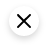• Machine Learning Decision Tree – Solved Problem (ID3 algorithm)
• Poisson Distribution | Probability and Stochastic Process
• Conditional Probability | Joint Probability
• Solved assignment problems in communicaion |online Request
• while Loop in C++

## EngineersTutor

Fourier transform solved problems | signals & systems.• ← Fourier transform properties | Part 2 | Signals & Systems
• DTFT | Discrete Time Fourier Transform →

## Gopal Krishna

Hey Engineers, welcome to the award-winning blog,Engineers Tutor. I'm Gopal Krishna. a professional engineer & blogger from Andhra Pradesh, India. Notes and Video Materials for Engineering in Electronics, Communications and Computer Science subjects are added. "A blog to support Electronics, Electrical communication and computer students".## You May Also Like

Deterministic and random signals | signals & systems, fourier transformation of signals | signals and systems, even and odd signals | signal & systems, leave a reply cancel reply.

## Fourier Transform Examples

Here we will learn about Fourier transform with examples.

The Fourier transform of $f(x)$ is denoted by $\mathscr{F}\{f(x)\}= $$F(k), k \in \mathbb{R}, and defined by the integral : \mathscr{F}\{f(x)\}=F(k)=\frac{1}{\sqrt{2 \pi}} \int_{-\infty}^{\infty} e^{-i k x} f(x) d x Where \mathscr{F} is called fourier transform operator . The inverse Fourier transform, denoted by \mathscr{F}^{-1}\{F(k)\}=f(x), is defined by \mathscr{F}^{-1}\{F(k)\}=f(x)=\frac{1}{\sqrt{2 \pi}} \int_{-\infty}^{\infty} e^{i k x} F(k) d k where \mathscr{F}^{-1} is called the inverse Fourier transform operator . ## Examples of Fourier Transform Find the fourier transform of exp \left(-a x^{2}\right) .. By fourier transform formula we have, F(k)=\frac{1}{\sqrt{2 \pi}} \int_{-\infty}^{\infty} e^{-i k x-a x^{2}} d x =\frac{1}{\sqrt{2 \pi}} \int_{-\infty}^{\infty} \exp \left[-a\left(x+\frac{i k}{2 a}\right)^{2}-\frac{k^{2}}{4 a}\right] d x =\frac{1}{\sqrt{2 \pi}} \exp \left(-k^{2} / 4 a\right) \int_{-\infty}^{\infty} e^{-a y^{2}} d y =\frac{1}{\sqrt{2 a}} \exp \left(-\frac{k^{2}}{4 a}\right) Here is the graph of fourier transform## Find the Fourier transform of a below non periodic function f(x)=\left\{\begin{array}{ll}{1,} & {-1\lt x\lt 1} \\ {0,} & {|x|\gt 1}\end{array}\right.The above function is not a periodic function. A non periodic function cannot be represented as fourier series.But can be represented as Fourier integral . Then,using Fourier integral formula we get, F(k)=\frac{1}{2 \pi} \int_{-\infty}^{\infty} f(x) e^{-i k x} d x =\frac{1}{2 \pi} \int_{-1}^{1} e^{-i k x} d x =\frac{1}{2 \pi}\left.\frac{e^{-i k x}}{-i k}\right|_{-1} ^{1}$$ =\frac{1}{\pi k} \frac{e^{-i k}-e^{i k}}{-2 i}$$=\frac{\sin k}{\pi k}$

This is the Fourier transform of above function.

We can find Fourier integral representation of above function using fourier inverse transform .

$f(x)=\int_{-\infty}^{\infty} \frac{\sin k}{\pi k} e^{i k x} d x$ $=\frac{1}{\pi} \int_{-\infty}^{\infty} \frac{\sin k(\cos k x+i \sin k x}{k} d k$ $=\frac{2}{\pi} \int_{0}^{\infty} \frac{\sin k \cos k x}{k} d k$

This is the fourier integral representation of our non periodic function.

• Wikipedia Fourier Transform
• Geometric series
• Integration by parts
• For educators
• Greek Alphabet

## This problem has been solved!

You'll get a detailed solution from a subject matter expert that helps you learn core concepts.

## Question: What is the Fourier transform of 2cos(10t+3)?

General guidence

Step by step solution given below## Not the question you’re looking for?

Post any question and get expert help quickly.#### IMAGES

1. Fourier Transform (Solved Problem 7)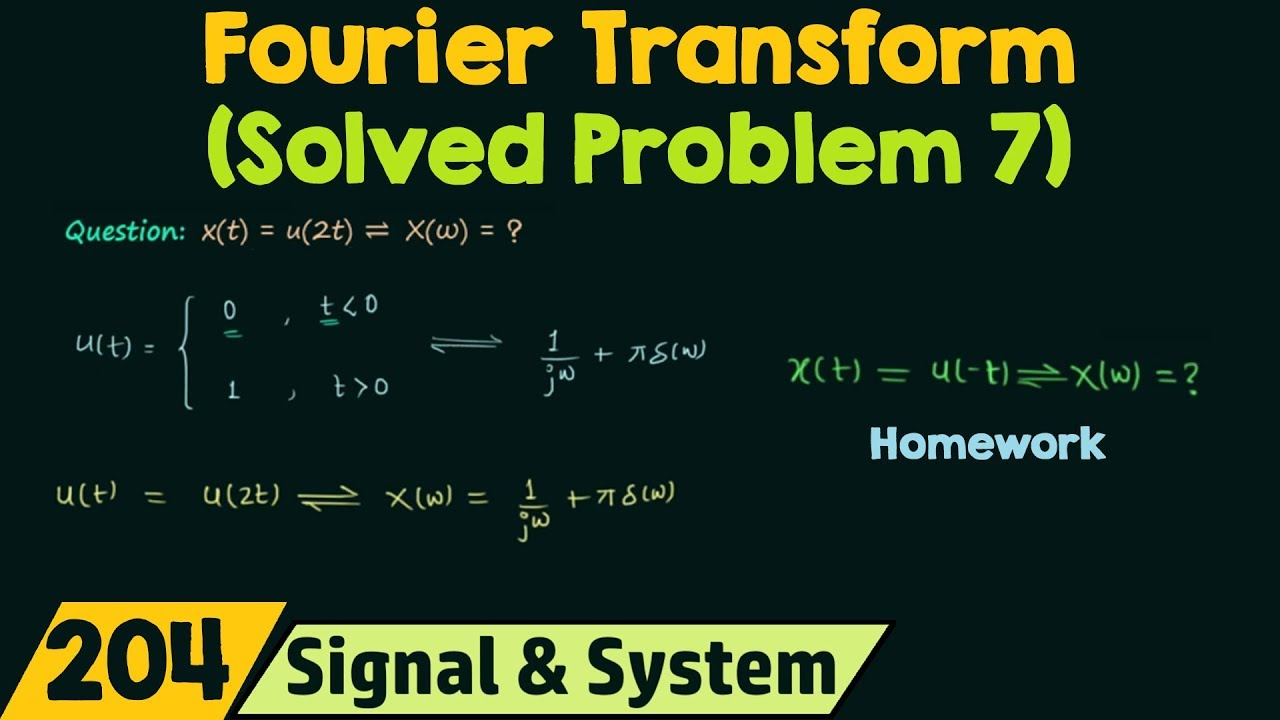2. fourier transform example problems and solutions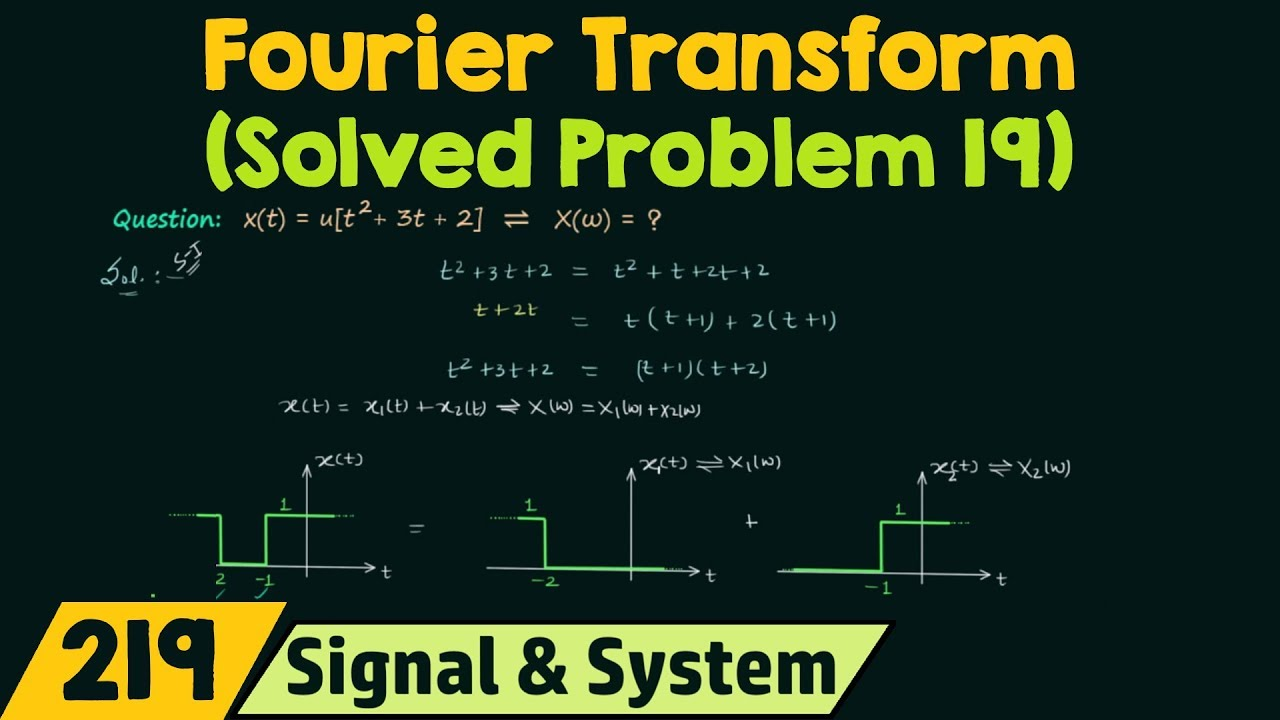3. fourier transform example problems and solutions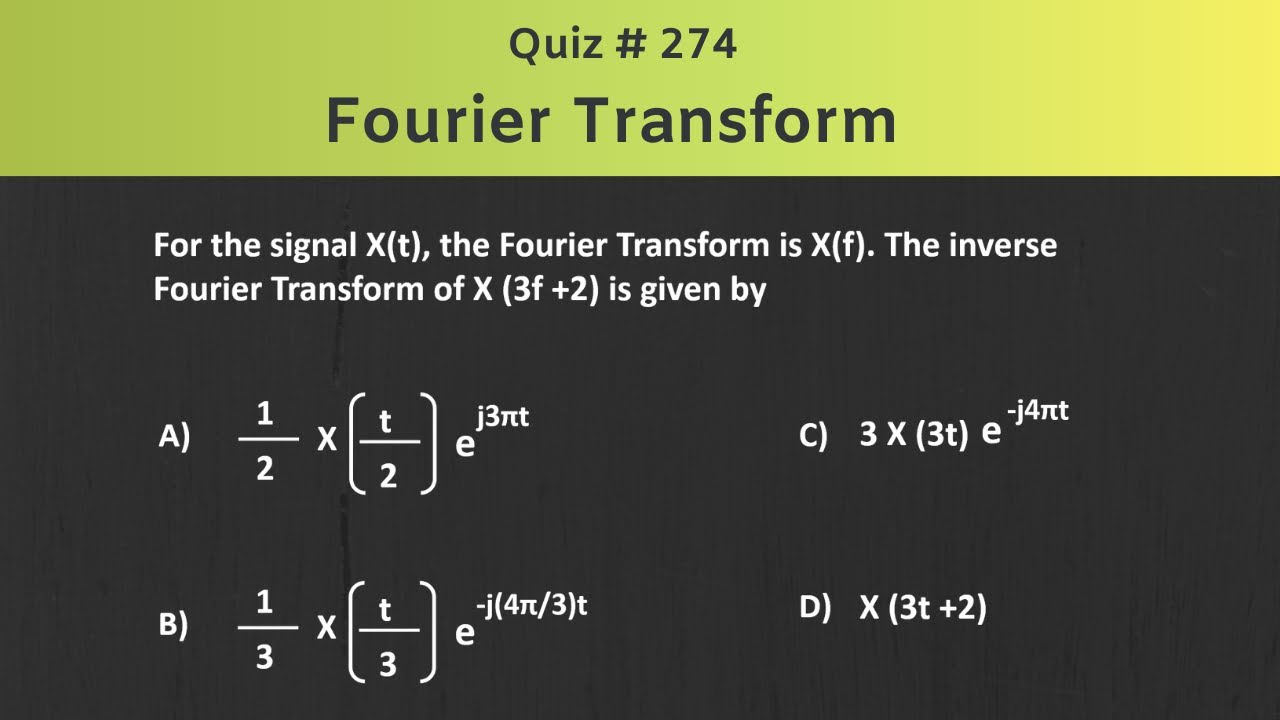4. Fourier Transform (Solved Problem 1)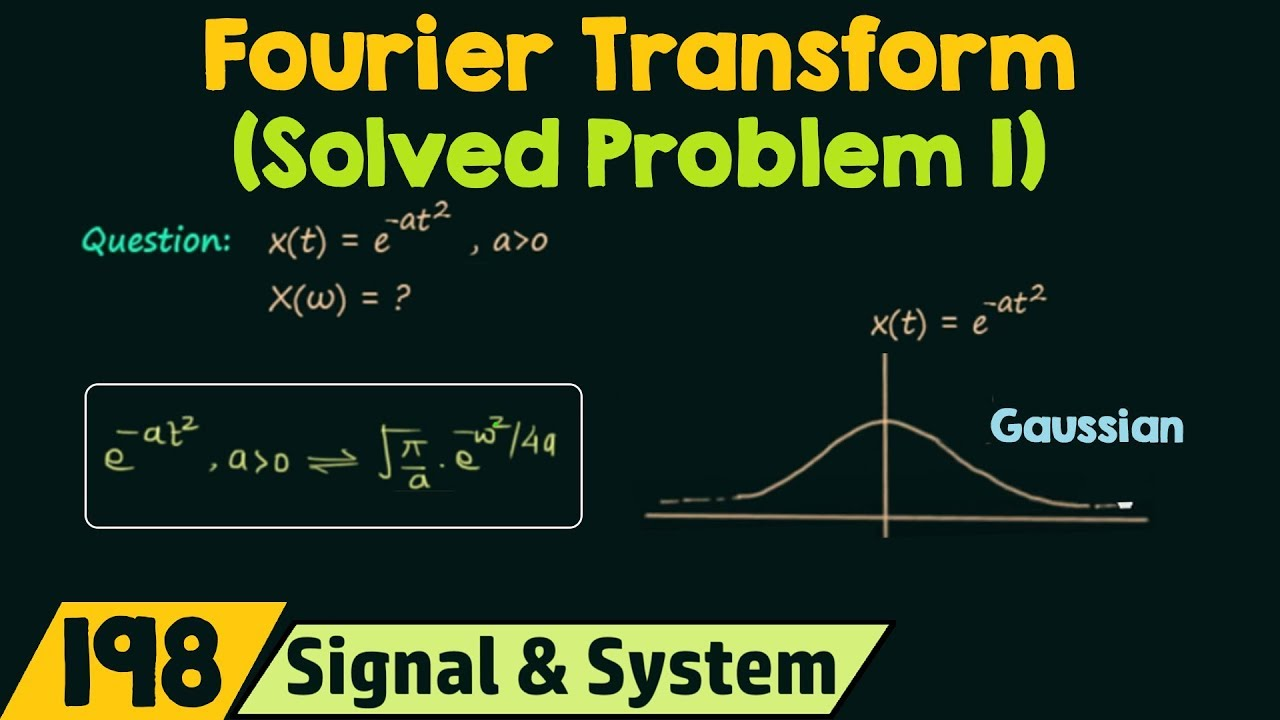5. Fourier transform examples and solutions || problem 4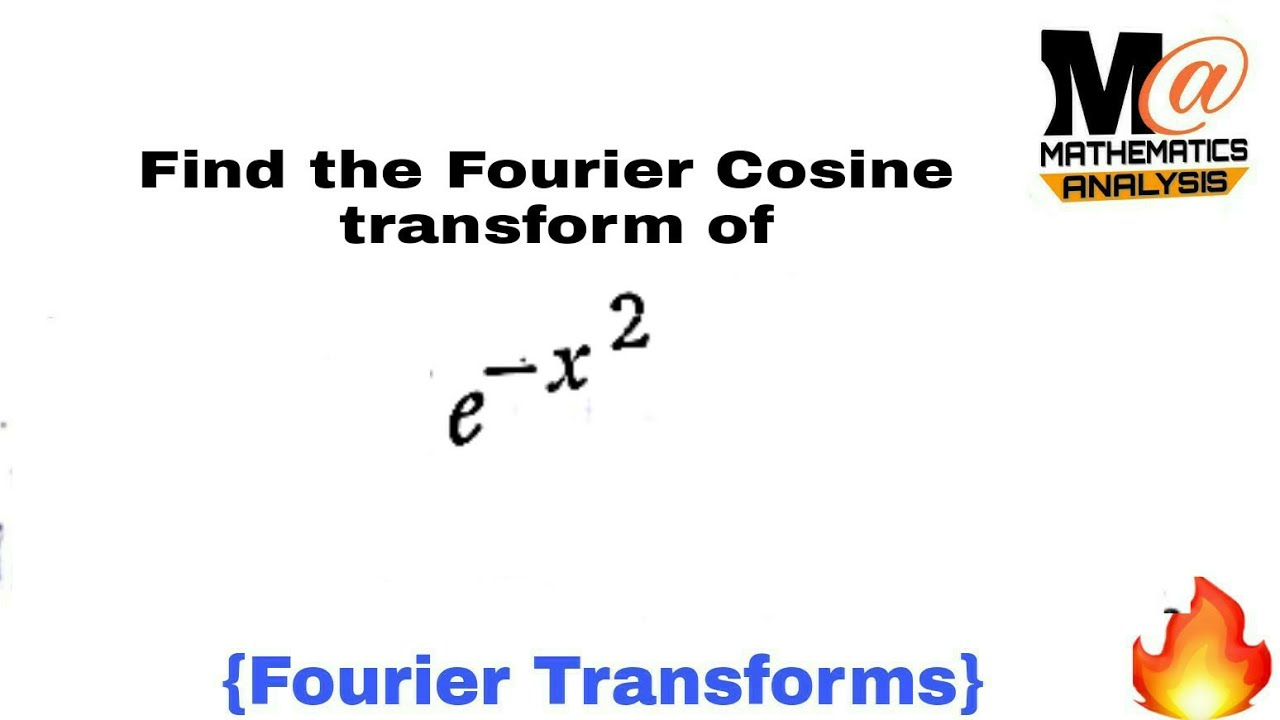6. Fourier transform solved problems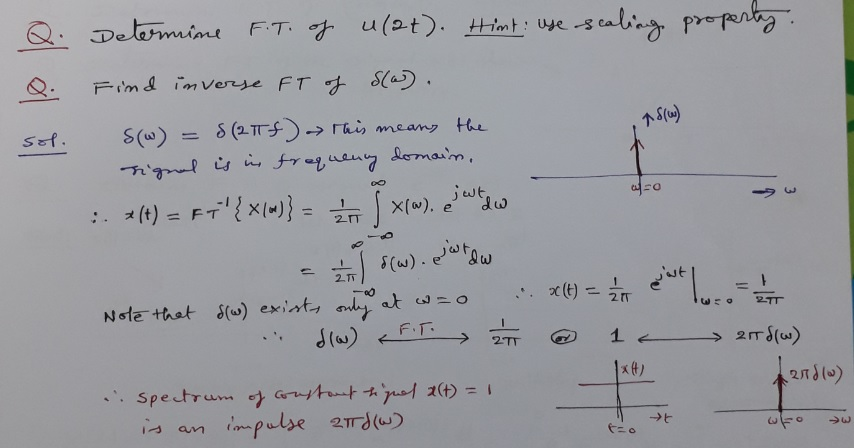#### VIDEO

1. Fourier transform examples and solutions || problem 5

2. PROPERTIES OF FOURIER TRANSFORM

3. FOURIER SERIES-PROBLEMS

4. Properties of Fourier Transform

5. 5 1 Properties of the Fourier Transform

6. Fourier transform examples and solutions || problem 4

1. PDF Problems and solutions for Fourier transforms and -functions

Since the F.T. of a convolution is a product, the F.T. of a triangle function (convolution of top hats) is the square of the F.T. of a single top hat of half the width. The result of qu. 2(b) thus follows from that of 2(a). 6. Prove the following results for delta functions.

2. PDF Fourier Transforms

Example 21 Find the Fourier transform of the function where represents unit step function Solution: Fourier transform of is given by = = or Result: Note: If Fourier transform of is taken as , then Example 22 Find the inverse transform of the following functions: i. ii. iii.

3. PDF 6 Fourier transform

!) 1 F sin( if jxj < > < (x) = 1 if jxj = ! 2 : 0 otherwise: Question 105: Use the Fourier transform technique to solve the following PDE: @tu(x; t) + c@xu(x; t) + u(x; t) = 0; for all x 2 (1 ; +1), t > 0, with u(x; 0) = u0(x) for all x 2 (1 ; +1). Solution: By taking the Fourier transform of the PDE, one obtains

4. 9.5: Properties of the Fourier Transform

This function is called the box function, or gate function. It is shown in Figure 9.5.3. The Fourier transform of the box function is relatively easy to compute. It is given by ˆf(k) = ∫∞ − ∞f(x)eikxdx = ∫a − abeikxdx = b ikeikx|a − a = 2b k sinka. We can rewrite this as ˆf(k) = 2absinka ka ≡ 2absinc ka.

5. PDF Lecture 8: Fourier transforms

Matthew Schwartz Lecture 8: Fourier transforms 1 Strings To understand sound, we need to know more than just which notes are played - we need the shape of the notes. If a string were a pure infinitely thin oscillator, with no damping, it would produce pure notes.

6. PDF 18.03 Practice Problems on Fourier Series { Solutions

18.03 Practice Problems on Fourier Series { Solutions Graphs appear at the end. 1. What is the Fourier series for 1 + sin2 t? This function is periodic (of period 2 ), so it has a unique expression as a Fourier series. It's easy to nd using a trig identity. By the double angle formula, cos(2t) = 1 3 1 + sin2 t = 2 1 cos(2t): 2 2 sin2 t, so

7. PDF Fourier Analysis Notes, Spring 2020

The rst part of the course discussed the basic theory of Fourier series and Fourier transforms, with the main application to nding solutions of the heat equation, the Schr odinger equation and Laplace's equation. For the Fourier series, we roughly followed chapters 2, 3 and 4 of , for the Fourier transform, sections 5.1 and 5.2 .

8. PDF 8 Continuous-Time Fourier Transform

Solutions to Recommended Problems S8.1 x(t) Tj Tj 2 2 Figure S8.1-1 Note that the total width is T,. (b) i(t) 2 3T1 --T1 To 2 T1 2 T1 To 2 2 Figure S8.1-2 Tl 3T =O (c) Using the definition of the Fourier transform, we have X(w) = ox(t)e -j dt = Ti/2 le-j" dt since x(t) = 0 for Til 12 = - (e -jwTI1/2 _ eT1/2) JW

9. Assignments

This section contains recommended problems and solutions. Browse Course Material Introduction Readings ... Continuous-time Fourier transform 9 Fourier transform properties 10 Discrete-time Fourier series 11 Discrete-time Fourier transform ... Feedback example: The inverted pendulum Course Info Instructor Prof. Alan V. Oppenheim ...

10. PDF 10 Discrete-Time Fourier Series

Similarly, the Fourier series of this sequence is given by ak = 5 -1 1 + cos (5 , for all k This result can also be obtained by using the fact that the Fourier series coeffi­ cients are proportional to equally spaced samples of the discrete-time Fourier transform of one period (see Section 5.4.1 of the text, page 314). S10.5

11. Chapter 9: Fourier Transform

The damped cosine wave has a spread in time that is measured by the half-width $\Delta t = 1/\kappa$, which marks the point at which the wave's envelope has decreased by a factor of $1/e \simeq 0.4$.

12. PDF Fourier Transform Examples

M M If f(x) decays fast enough as x!1and x!1 , then fb(w) is also de ned. However there are much larger collections of objects for which the transform can be de ned. For example, if (x) is the Dirac delta function, then b(w) = 1= p 2ˇthe constant function. Also one can see that the inverse transform of (w) is the constant function 1= p 2ˇ.

13. PDF Twenty Questions on the Fourier Transform

Find the Fourier Transform of each signal without doing any integration. (Use table-lookup and tricks.) A(t)=e−atu(t−k);a>0;B(t)=t7e−atu(t);a>0;C(t)= eiWt a2+t2 ; D(t)=t[u(t)−u(t−k)];E(t)= sin(2t)−3cos(2t) t2+25 ;F(t)= XN k=0 a k dk dtk y(t): G(t)= X1 k=−1 e−jt−2kj;H(t)= X1 k=0 k (t−k); j j<1;I(t)=te−2tsin(4t)u(t): 4.

14. PDF 9 Fourier Transform Properties

Fourier Transform Properties / Problems P9-5 (a) Show that the left-hand side of the equation has a Fourier transform that can be expressed as A(w)Y(w), where Y(w) = J{y(t)} Find A(w). (b) Similarly, show that the right-hand side of the equation has a Fourier transform that can be expressed as ...

15. PDF Chapter10: Fourier Transform Solutions of PDEs

Φ(x) = c1 cos λx + c2 sin λx (8) which remains bounded at x = ±∞ for all λ > 0. The major difference from the finite domain problem is that in this case all λ > 0 are eigenvalues! Q: Show that λ = 0 is an eigenvalue of the problem (6),(7) and the corresponding eigenfunction is Φ(x) ≡ c.

16. 5.E: Fourier Transform (Exercises)

Integrated by Justin Marshall. 5.E: Fourier Transform (Exercises) is shared under a not declared license and was authored, remixed, and/or curated by LibreTexts. These are homework exercises to accompany Miersemann's "Partial Differential Equations" Textmap. This is a textbook targeted for a one semester first course on differential equations ...

17. PDF Chapter 1 The Fourier Transform

The Fourier Transform 1.1 Fourier transforms as integrals There are several ways to de ne the Fourier transform of a function f: R ! C. In this section, we de ne it using an integral representation and state some basic uniqueness and inversion properties, without proof. Thereafter, we will consider the transform as being de ned as a suitable ...

18. CT Fourier transform practice problems list

Collectively solved problems on continuous-time Fourier transform. Computation of CT Fourier transform. Compute the Fourier transform of e^-t u (t) Compute the Fourier transform of cos (2 pi t). Compute the Fourier transform of cos (2 pi t + pi/12). Compute the Fourier transform of a rectangular pulse-train. Compute the Fourier transform of a ...

19. 29: Solving the Wave Equation with Fourier Transforms

The Fourier transform is 1 where k = 2 and 0 otherwise. We see that over time, the amplitude of this wave oscillates with cos(2 v t). The solution to the wave equation for these initial conditions is therefore $$\Psi (x, t) = \sin ( 2 x) \cos (2 v t)$$. This wave and its Fourier transform are shown below.

20. 9.11: Transforms and Partial Differential Equations

Fourier Transform and the Heat Equation. We will first consider the solution of the heat equation on an infinite interval using Fourier transforms. The basic scheme has been discussed earlier and is outlined in Figure $$\PageIndex{1}$$. Figure $$\PageIndex{1}$$: Using Fourier transforms to solve a linear partial differential equation.

21. Fourier Transform

The Fourier transform is a type of mathematical function that splits a waveform, which is a time function, into the type of frequencies that it is made of. The result generated by the Fourier transform is always a complex-valued frequency function. The Fourier transform's absolute value shows the frequency value existing in the original ...

22. Fourier transform solved problems

October 26, 2018 Gopal Krishna 15309 Views 0 Comments fourier transform solved problems. Hey Engineers, welcome to the award-winning blog,Engineers Tutor. I'm Gopal Krishna. a professional engineer & blogger from Andhra Pradesh, India. Notes and Video Materials for Engineering in Electronics, Communications and Computer Science subjects are ...

23. Fourier Transform example : All important fourier transforms

Example #1 Find the Fourier transform of exp . By fourier transform formula we have, Here is the graph of fourier transform Example #2 Find the Fourier transform of a below non periodic function The above function is not a periodic function. A non periodic function cannot be represented as fourier series.But can be represented as Fourier integral.

24. Question: What is the Fourier transform of 2cos(10t+3)?

Expert Answer. Step 1. General guidence. Step by step solution given below. View the full answer. Step 2.